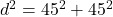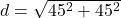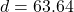## Two cars depart an intersection. One goes north. The other goes west. Each car travels at a constant speed of 30 miles per

Question

Two cars depart an intersection.
One goes north.
The other goes west.
Each car travels at a constant speed of 30 miles per hour.
Assuming the roads go on forever, what will be the distance between the cars after 1 hours and 30 minutes in miles?

in progress 0
5 months 2021-08-18T08:33:01+00:00 1 Answers 1 views 0

The distance between them after 1 hour and 30 minutes will be of 63.64 miles.

Step-by-step explanation:

This question can be solved using the Pythagorean Theorem.

Both cars travel at a constant speed of 30 miles per hour. So, after 1 hour and 30 minutes, which is 1 and a half hour, they will have traveled 30*1.5 = 45 miles.

The distance between them is the hypotenuse of a right triangle, in which the sides are 45. SoThe distance between them after 1 hour and 30 minutes will be of 63.64 miles.